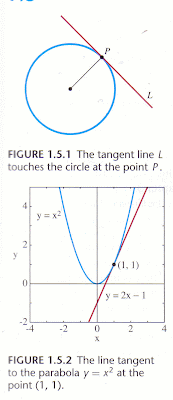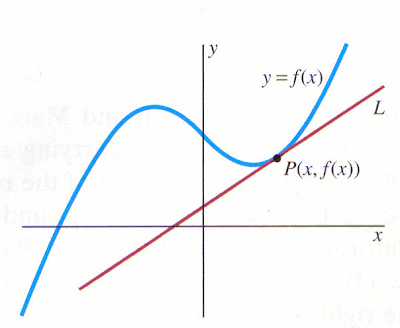## 25 Jul 2012

### Preview: What Is Calculus? in Ewards and Penney's Calculus

presentation of Edwards & Penney's work, by Corry Shores
[Search Blog Here. Index-tags are found on the bottom of the left column.]

[Central Entry Directory]
[Mathematics, Calculus, Geometry, Entry Directory]
[Calculus Entry Directory]
[Edwards & Penney, Entry Directory]

Edwards & Penney's Calculus is an incredibly-impressive, comprehensive, and understandable book. I highly recommend it.

[Search Blog Here. Index-tags are found on the bottom of the left column.]

[I your author am not a mathematician; I am merely an admirer of Edwards & Penney's wonderful calculus book. Please consult the text or other references to be certain about anything in the summary below. I mean this emphatically.]

[This section introduces problems that we later discuss much further in detail.]

Summary of
Edwards & Penney
Calculus

Chapter 1. Functions, Graphs, and Models
Section 1.5. Preview: What Is Calculus?

Calculus ("the calculus") is a body of computational techniques. It revolves around two basic geometrical problems, and mathematicians have been dealing with these problems for over 2000 years. Both problems involve "the graph y = f(x) of a given function." (p.45)

Problem 1: The Tangent Problem

One problem calculus tries to solve is finding the 'line tangent' at a given point on a curve y = f(x).Edwards and Penney then formulate the tangent problem with reference to this graph:So let's first recall what a function is. Functions describe relationships between variables. The function describes the way that one variable varies with respect to another variable, often formulated y = f(x). So consider the function y = x2.We have two variables, x and y. Both are varying, both increase in value. But the way that the one increases with respect to the other is further describable. As the x value increases, the y value increases to the power of two. So when x has the value of 2, y has the value of 4. And when x is 4, y is 16, and so on. Edwards and Penney offer this definition for functions.

Function:
A real-valued function f defined on a set D of real numbers is a rule that assigns to each number x in D exactly one real number, denoted by f (x).

The set D of all numbers for which f (x) is defined is called the domain (or domain of definition) of the function f. The number f (x), read "f of x," is called the value of the function f at the number (or point) x. The set of all values y = f (x) is called the range of f . That is, the range of f is the set {y: y = f (x) for some x in D). [p.2d]

So recall again the graph Edwards and Penney are using to describe the tangent problem.Here we see that x varies with respect to y in such a way that the series of their correlated variations makes a waving curve. Now we consider a point P along the continuous variation of x's and y's correlations. a point is described with the x and y coordinate values (x, y). But the way we find y is by finding the value of the function applied to the value of x. So the y value can also be noted as f(x), and thus the coordinates for point P along the function y = f(x) would be P(x, f(x)). In the case of y = x2, we might consider the point (2, 4) for example. Thus the tangent problem:

The Tangent Problem
Given a point P(x, f(x)) on the curve y = f(x), how do we calculate the slope of the tangent line at P?

Finding the tangent gives us what is called the derivative of the function f, and later Edwards and Penney explain how we obtain derivatives. This is largely a matter of differential calculus.

ExampleWe are driving down a straight road. It takes us a certain amount of time (t) to go a certain distance, and the way that the distance increases as time increases can be described with the function y = f(t). If we find the slope at point (t, f(t)), then we find the velocity at time t.

Problem 2: The Area Problem

Consider this graph.We might want to know what the area is below the curve between a and b.

The Area Problem
If f(x) ≧ 0 for x in the interval [a, b], how do we calculate the area A of the plane region that lies between the curve y = f(x) and the x-axis over the interval [a, b]?

Example

Consider this graph.Unlike the prior example which correlated the way that distance varied with respect to time, this new graph shows how velocity varies with respect to time, given with the function y = f(t). So f(t) gives us the velocity of the car at time t. This means that the area under the curve within the time interval [a, b] gives us the distance the car travels between time a and time b.

Text summary and images from:
Edwards & Penney: Calculus. New Jersey: Prentice Hall, 2002, pp.2; 45-47.